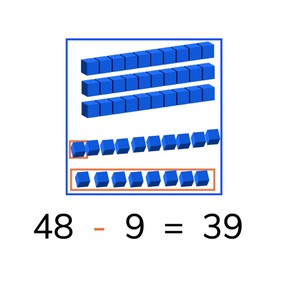Subtraction to 50 with subtrahends <10

# Subtraction to 50 with subtrahends <10

I can subtract numbers to 10 from numbers to 50.8,000 schools use Gynzy92,000 teachers use Gynzy1,600,000 students use Gynzy

## General

Students learn to subtract numbers to 50 with minuends less than 10.

2.NBT.B.5

## Relevance

It is important for students to learn to subtract numbers to 50 so they can determine how many are left of larger quantities.

## Introduction

Start by practicing with a number line to 50 by asking students to write a number from 1 to 50 on a sheet of paper. Then they must form a number line as a class without talking! Then ask students to solve the subtraction problem given on a number line to 30 with a minuend greater than ten.

## Development

Check that students are able to subtract numbers to 50 by asking the following questions:
- Why is it useful to be able to subtract numbers to 50?
- How do you solve a problem like 45-7?

## Guided practice

Students start with subtraction problems with visual support, then in the abstract and then practice story problems.

## Closing

Ask students to do a visual, abstract, and story subtraction problem. You may have them solve these in groups or pairs.

## Teaching tips

You can offer manipulatives such as blocks or a number line to help students solve subtraction problems to 50.

## Instruction materials

Optional: blocks or number lines

### The online teaching platform for interactive whiteboards and displays in schools

• Save time building lessons

• Manage the classroom more efficiently

• Increase student engagement